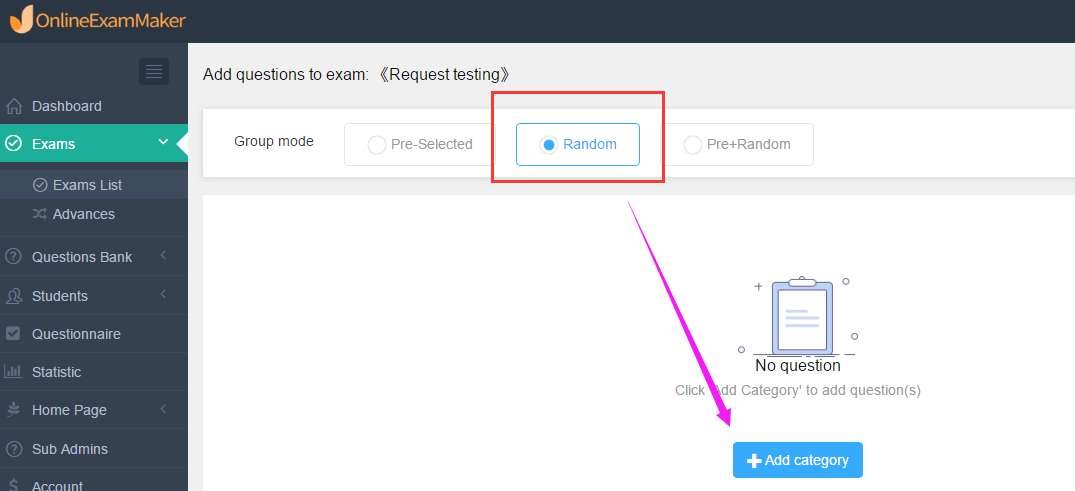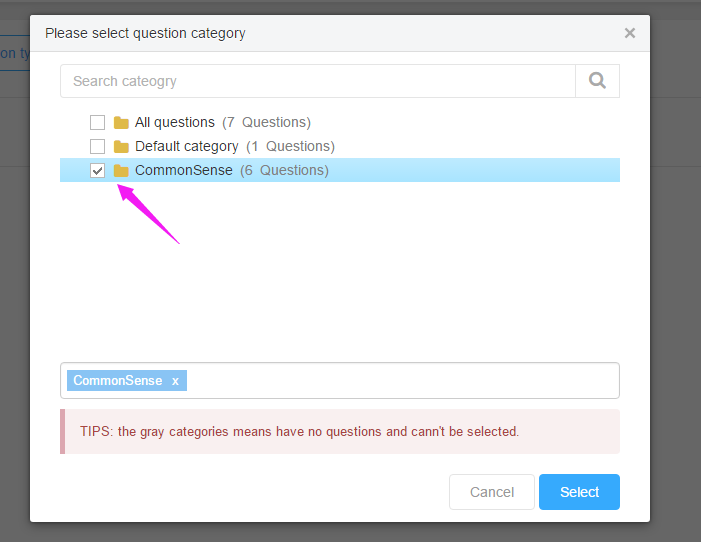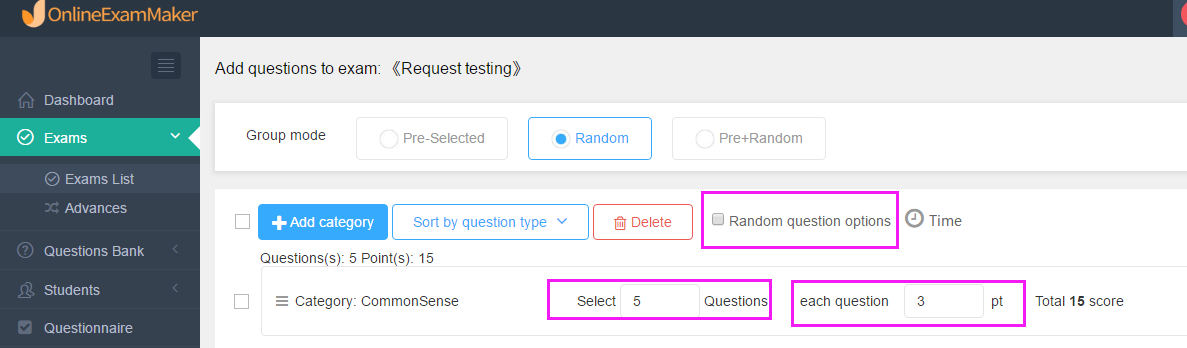## Does online exam system support to select questions at random from question bank to build up an exam?

Does online exam system support to select questions at random from question bank to build up an exam? This is not a problem now. If we want to make the test questions different for each candidate, we can achieve it through the online exam system.

Now, our users will use our online exam maker to create online exam by select questions at random from question bank to make each candidate’s question different, which can also effectively prevent them from cheating.

Let’s see how to  select questions at random from a question bank each time the exam is taken by using our software?

As what can be seen in the picture below, our online exam maker  has multiple modes of forming online exam papers. If you want to select questions at random from a question bank each time the exam is taken,  then choose the Random mode  in the second step of creating the test paper, that is, the step of adding questions. (Click here for the tutorial of creating a exam; if you don’t have an account, sign up to get one. )Then, we  choose one or more question categoies to add questions;We can set the number of questions selected for each question type and the score of each question; as for the Multiple choice question, we can make the options random.Next we complete the other settings of the exam paper, and then publish the test paper link. When the candidate opens the exam, the system will randomly present questions!